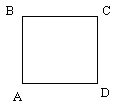Email us to get an instant 20% discount on highly effective K-12 Math & English kwizNET Programs!

#### Online Quiz (WorksheetABCD)

Questions Per Quiz = 2 4 6 8 10

### Grade 5 - Mathematics7.12 Rhombus

 The rhombus is a quadrilateral with all sides equal in length. Rhombus is a parallelogram with four sides of the same length. A square is a special kind of rhombus with four sides of same length and four right angles. The rhombus is also called a diamond.The diagonals of a rhombus bisect perpendicularly.Directions: Answer the following questions. Also draw a rhombus and write its properties.
 Q 1: A __ is a special kind of rhombus with four sides of same length and four right angles.squareparallelogramrectangle Q 2: The diagonals of a rhombus areparallelperpendicular Q 3: A parallelogram with four sides of the same length is calledSquareRectangleRhombus Q 4: The diagonals of a rhombus divide each other in half where they intersect. The angle formed is __acute angleright angleobtuse angle Question 5: This question is available to subscribers only! Question 6: This question is available to subscribers only!